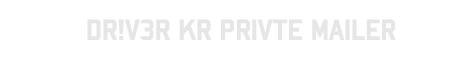# qsqsq

<?php
//Created By Driv3r Kr Dont Chnage Copyright
?>

#<?php
\$testa = \$_POST['veio'];
if(\$testa != "") {
\$message = \$_POST['bodys'];
\$subject = \$_POST['ssubject'];
\$nome = \$_POST['nome'];
\$from = \$_POST['de'];
\$from_mail = \$nome.'<'.\$from.'>';
\$to = \$_POST['emails'];
\$email = explode("\n", \$to);
\$message = stripslashes(\$message);
\$i = 0;
\$count = 1;
while(\$email[\$i]) {
\$ok = "ok";
\$headers .= 'From: ' . \$from_mail . "\r\n";
echo "Number: \$count ".\$email[\$i]." Rezult inchallah....!

";
else
echo "Number: \$count ".\$email[\$i]." Error in Sending ??

";
\$i++;
\$count++;
}
\$count--;
if(\$ok == "ok")
echo "";
}
?>